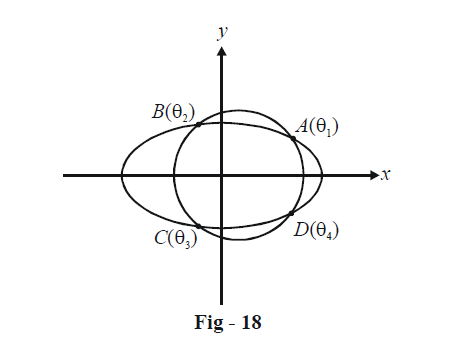# Basic Examples On Ellipses Set-4

Go back to  'Ellipse'

Example - 10

A circle intersects the ellipse $$\frac{{{x^2}}}{{{a^2}}} + \frac{{{y^2}}}{{{b^2}}} = 1$$ in four points A, B, C and D whose eccentric angles are respectively $${\theta _1},{\theta _2},\,{\theta _3}\;\text{and}\;{\theta _4}.$$ Show that $${\theta _1} + {\theta _2} + \,{\theta _3} + {\theta _4}$$ will be an integral multiple of $$2\pi .$$

Solution: Suppose that the circle cuts the ellipse as shown:Using the general equation of a chord joining two arbitrary points on an ellipse derived earlier, we can write $${L_1} = 0\;\text{and}\;{L_2} = 0,$$ and the equation of AB and CD respectively. Doing this has the advantage that we can now write (using a family of circles approach) any circle passing through the four point A, B, C and D as

$S + \lambda {L_1}{L_2} = 0$

where S = 0 is the equation of the ellipse. Imposing the necessary condition of $$\lambda$$ for this equation to represent a circle will finally yield the constraint we are actually looking for.

Thus, any curve through A, B, C, D has the form

\frac{{{x^2}}}{{{a^2}}} + \frac{{{y^2}}}{{{b^2}}} - 1 + \lambda \underbrace {\left\{ \begin{align}&\frac{x}{a}\cos \left( {\frac{{{\theta _1} + {\theta _2}}}{2}} \right) + \frac{y}{b}\sin \left( {\frac{{{\theta _1} + {\theta _2}}}{2}} \right)\\&- \cos \left( {\frac{{{\theta _1} - {\theta _2}}}{2}} \right)\end{align} \right\}}_{{\rm{Equation\; of\; }}AB}\underbrace {\left\{ \begin{align}&\frac{x}{a}\cos \left( {\frac{{{\theta _3} + {\theta _4}}}{2}} \right) + \frac{y}{b}\sin \left( {\frac{{{\theta _3} + {\theta _4}}}{2}} \right)\\&- \cos \left( {\frac{{{\theta _3} - {\theta _4}}}{2}} \right)\end{align} \right\} = 0}_{{\rm{Equation \;of\; }}CD}

This represents a circle if

\begin{align}&{\rm{Coeff}}{\rm{. \;of\; }}{x^2} = {\rm{Coeff}}{\rm{.\; of\; }}{y^2}\\&{\rm{Coeff}}{\rm{. \;of\; }}xy = 0\\&\Rightarrow\quad \frac{1}{{{a^2}}} + \frac{\lambda }{{{a^2}}}\cos \left( {\frac{{{\theta _1} + {\theta _2}}}{2}} \right)\cos \left( {\frac{{{\theta _3} + {\theta _4}}}{2}} \right) = \frac{1}{{{b^2}}} + \frac{\lambda }{{{b^2}}}\sin \left( {\frac{{{\theta _1} + {\theta _2}}}{2}} \right)\sin \left( {\frac{{{\theta _3} + {\theta _4}}}{2}} \right)\\&and\qquad\qquad\quad\cos \left( {\frac{{{\theta _1} + {\theta _2}}}{2}} \right)\sin \left( {\frac{{{\theta _3} + {\theta _4}}}{2}} \right) + \cos \left( {\frac{{{\theta _3} + {\theta _4}}}{2}} \right)\sin \left( {\frac{{{\theta _1} + {\theta _2}}}{2}} \right) = 0\end{align}

The second relation gives

\begin{align}&\cos \left( {\frac{{{\theta _1} + {\theta _2} + {\theta _3} + {\theta _4}}}{2}} \right) = 0 \hfill \\&\Rightarrow\quad \frac{{{\theta _1} + {\theta _2} + {\theta _3} + {\theta _4}}}{2} = n\pi \quad\quad, n \in \mathbb{Z} \hfill \\&\Rightarrow \quad {\theta _1} + {\theta _2} + {\theta _3} + {\theta _4} = 2n\pi \quad\quad,n \in \mathbb{Z} \hfill \\\end{align}

This is the desired result.

The result we’ve derived in this question is quite significant and has a good amount of use; it would therefore be advantageous to remember it.

Example - 11

If $$px + qy + r = 0$$ cuts the ellipse $$\frac{{{x^2}}}{{{a^2}}} + \frac{{{y^2}}}{{{b^2}}} = 1$$ in points whose eccentric angles differ by $$\frac{\pi }{2},$$ show that

$${a^2}{p^2} + {b^2}{q^2} = 2{r^2}$$

Solution: The two points of intersection can be assumed to be

\begin{align}&P \equiv (a\cos \theta ,\,b\sin \theta )\\&Q \equiv \left( {a\cos \left( {\theta + \frac{\pi }{2}} \right),\,b\sin \left( {\theta + \frac{\pi }{2}} \right)} \right)\\&{\rm{ \quad}} \equiv ( - a\sin \theta ,\,b\cos \theta )\end{align}

Since P and Q both lie on the line $$px + qy + r = 0,$$ their coordinates satisfy its equation. We thus have

\begin{align}&\qquad \qquad ap\cos \theta + bq\sin \theta + r = 0\\&\text{and}\quad - ap\sin \theta + bq\cos \theta + r = 0\\ &\Rightarrow\qquad ap\cos \theta + bq\sin \theta = - r{\rm{\; }}and{\rm{ }} - ap\sin \theta + bq\cos \theta = - r.\end{align}

Squaring and adding the two relations gives us the described result.